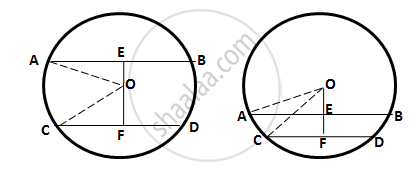Share

Books Shortlist

# In a Circle of Radius 17 Cm, Two Parallel Chords of Lengths 30 Cm and 16 Cm Are Drawn. Find the Distance Between the Chords, If Both the Chords Are (I) on the Opposite Sides of the Centre, - ICSE Class 10 - Mathematics

ConceptChord Properties - the Perpendicular to a Chord from the Center Bisects the Chord (Without Proof)

#### Question

In a circle of radius 17 cm, two parallel chords of lengths 30 cm and 16 cm are drawn. Find the distance between the chords, if both the chords are
(i) on the opposite sides of the centre,
(ii) on the same side of the centre.

#### Solution

Let O be the centre of the circle and AB and CD be the two parallel chords of length 30 cm and 16 cm respectively. Drop OE and OF perpendicular on AB and CD from the centre O.OP⊥AB and OF⊥CD
∴ OE bisects AB and OF bisects CD
(Perpendicular drawn from the centre of a circle to a chord bisects it)

⇒ AE 30/2 =15cm ; CF = 16/2 = 8 cm

In right ∆OAE,

OA2 = OE2 + AE2

⇒ OE2 = OA2 - AE2 = (17)2 - (15)2 = 64
∴ OE = 8cm
In right ∆OCF,

OC2 = OF2 + CF2

⇒ OF2 = OC2 - CF2 = (17)2 - (8)2 = 225

∴ OF = 15 cm

(i) The chords are on the opposite sides of the centre:
∴ EF = EO + OF = (8 + 15) = 23 cm
(ii) The chords are on the same side of the centre:
∴ EF = OF - OE = (15 - 8) = 7 cm

Is there an error in this question or solution?

#### Video TutorialsVIEW ALL 

Solution In a Circle of Radius 17 Cm, Two Parallel Chords of Lengths 30 Cm and 16 Cm Are Drawn. Find the Distance Between the Chords, If Both the Chords Are (I) on the Opposite Sides of the Centre, Concept: Chord Properties - the Perpendicular to a Chord from the Center Bisects the Chord (Without Proof).
S